• +91 9971497814
• info@interviewmaterial.com

# Chapter 3 Trigonometric Functions Ex-3.1 Interview Questions Answers

### Related Subjects

Question 1 :
Find the radian measures corresponding to the following degree measures:
(i) 25° (ii) – 47° 30′ (iii) 240° (iv) 520°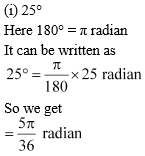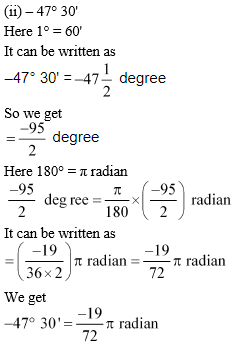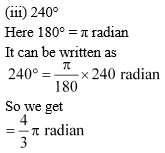(iv) 520°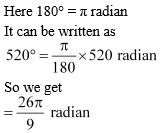Question 2 :
Find the degree measures corresponding to the following radian measures (Use π = 22/7)
(i) 11/16
(ii) -4
(iii) 5π/3
(iv) 7π/6

(i) 11/16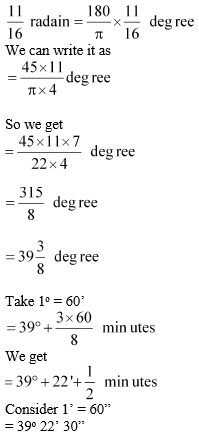(ii) -4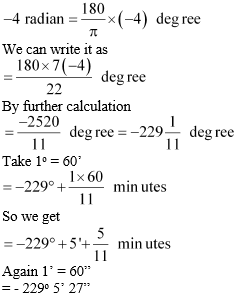(iii) 5π/3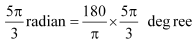We get

= 300o

(iv) 7π/6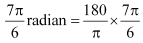We get

= 210o

Question 3 : A wheel makes 360 revolutions in one minute. Through how many radians does it turn in one second?

It is given that
No. of revolutions made by the wheel in
1 minute = 360
1 second = 360/60 = 6
We know that
The wheel turns an angle of 2π radian in one complete revolution.
In 6 complete revolutions, it will turn an angle of 6 × 2π radian = 12 π radian
Therefore, in one second, the wheel turns an angle of 12π radian.

Question 4 : Find the degree measure of the angle subtended at the centre of a circle of radius 100 cm by an arc of length 22 cm (Use π = 22/7).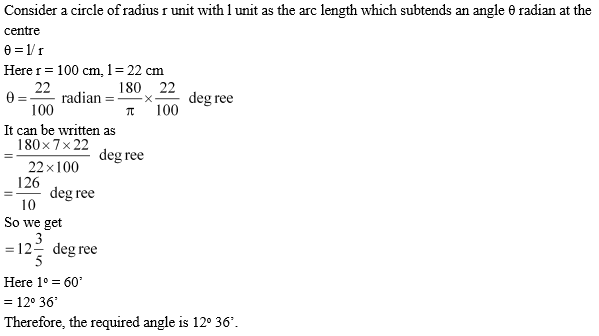Question 5 : In a circle of diameter 40 cm, the length of a chord is 20 cm. Find the length of minor arc of the chord.

The dimensions of the circle are
Diameter = 40 cm
Radius = 40/2 = 20 cm
Consider AB be as the chord of the circle i.e. length = 20 cm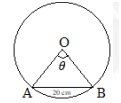In ΔOAB,
Radius of circle = OA = OB = 20 cm
Similarly AB = 20 cm
Hence, ΔOAB is an equilateral triangle.
θ = 60° = π/3 radian
In a circle of radius r unit, if an arc of length l unit subtends an angle θ radian at the centre
We get θ = 1/r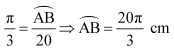Therefore, the length of the minor arc of the chord is 20π/3 cm.

Question 6 : If in two circles, arcs of the same length subtend angles 60° and 75° at the centre, find the ratio of their radii.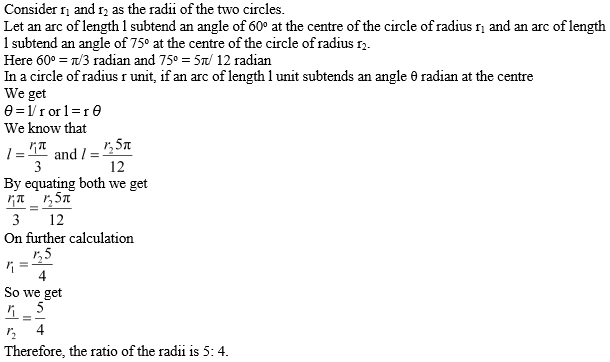Question 7 :
Find the angle in radian though which a pendulum swings if its length is 75 cm and the tip describes an arc of length
(i) 10 cm (ii) 15 cm (iii) 21 cm

In a circle of radius r unit, if an arc of length l unit subtends an angle θ radian at the centre, then θ = 1/r
We know that r = 75 cm
(i) l = 10 cm
So we get
By further simplification
(ii) l = 15 cm
So we get
By further simplification
(iii) l = 21 cm
So we get
By further simplification

Todays Deals### Chapter 3 Trigonometric Functions Ex-3.1 Contributorskrishan

Name:
Email:

# Latest News# 9000 interview questions in different categories